• 北京市
• 北京市
• 广东省
• 广东省
• 广州市
• 深圳市
• 珠海市
• 揭阳市
• 佛山市
• 肇庆市
• 潮州市
• 汕头市
• 阳江市
• 湛江市
• 韶关市
• 梅州市
• 河源市
• 惠州市
• 汕尾市
• 东莞市
• 中山市
• 云浮市
• 江门市
• 茂名市
• 清远市
• 辽宁省
• 辽宁省
• 沈阳市
• 大连市
• 鞍山市
• 抚顺市
• 本溪市
• 丹东市
• 锦州市
• 葫芦岛市
• 营口市
• 盘锦市
• 阜新市
• 辽阳市
• 铁岭市
• 朝阳市
• 吉林省
• 吉林省
• 长春市
• 吉林市
• 四平市
• 辽源市
• 通化市
• 白山市
• 松原市
• 白城市
• 延边朝鲜族自治州
• 黑龙江省
• 黑龙江省
• 哈尔滨市
• 齐齐哈尔市
• 鹤岗市
• 双鸭山市
• 鸡西市
• 大庆市
• 伊春市
• 牡丹江市
• 佳木斯市
• 七台河市
• 黑河市
• 绥化市
• 大兴安岭地区
• 上海市
• 上海市
• 黄浦区
• 卢湾区
• 徐汇区
• 长宁区
• 静安区
• 普陀区
• 闸北区
• 虹口区
• 杨浦区
• 闵行区
• 宝山区
• 嘉定区
• 浦东新区
• 金山区
• 松江区
• 青浦区
• 奉贤区
• 崇明县
• 江苏省
• 江苏省
• 南京市
• 徐州市
• 连云港市
• 淮安市
• 宿迁市
• 盐城市
• 扬州市
• 泰州市
• 南通市
• 镇江市
• 常州市
• 无锡市
• 苏州市
• 浙江省
• 浙江省
• 杭州市
• 宁波市
• 温州市
• 嘉兴市
• 湖州市
• 绍兴市
• 金华市
• 衢州市
• 舟山市
• 台州市
• 丽水市
• 安徽省
• 安徽省
• 合肥市
• 芜湖市
• 蚌埠市
• 淮南市
• 马鞍山市
• 淮北市
• 铜陵市
• 安庆市
• 黄山市
• 阜阳市
• 宿州市
• 巢湖市
• 六安市
• 亳州市
• 池州市
• 宣城市
• 滁州市
• 福建省
• 福建省
• 福州市
• 厦门市
• 三明市
• 莆田市
• 泉州市
• 漳州市
• 南平市
• 龙岩市
• 宁德市
• 江西省
• 江西省
• 南昌市
• 景德镇市
• 萍乡市
• 九江市
• 新余市
• 鹰潭市
• 赣州市
• 吉安市
• 宜春市
• 抚州市
• 上饶市
• 山东省
• 山东省
• 济南市
• 青岛市
• 淄博市
• 枣庄市
• 东营市
• 潍坊市
• 烟台市
• 威海市
• 济宁市
• 泰安市
• 日照市
• 莱芜市
• 临沂市
• 德州市
• 聊城市
• 滨州市
• 菏泽市
• 河南省
• 河南省
• 郑州市
• 开封市
• 洛阳市
• 平顶山市
• 焦作市
• 鹤壁市
• 新乡市
• 安阳市
• 濮阳市
• 许昌市
• 漯河市
• 三门峡市
• 南阳市
• 商丘市
• 信阳市
• 周口市
• 驻马店市
• 省直辖县级行政单位
• 湖北省
• 湖北省
• 武汉市
• 黄石市
• 襄樊市
• 十堰市
• 荆州市
• 宜昌市
• 荆门市
• 鄂州市
• 孝感市
• 黄冈市
• 咸宁市
• 随州市
• 恩施土家族苗族自治州
• 省直辖县级行政单位
• 湖南省
• 湖南省
• 长沙市
• 株洲市
• 湘潭市
• 衡阳市
• 邵阳市
• 岳阳市
• 常德市
• 张家界市
• 益阳市
• 郴州市
• 永州市
• 怀化市
• 娄底市
• 湘西土家族苗族自治州
• 广西壮族自治区
• 广西壮族自治区
• 南宁市
• 柳州市
• 桂林市
• 梧州市
• 北海市
• 防城港市
• 钦州市
• 贵港市
• 玉林市
• 百色市
• 贺州市
• 河池市
• 来宾市
• 崇左市
• 海南省
• 海南省
• 海口市
• 三亚市
• 省直辖行政单位
• 重庆市
• 重庆市
• 渝中区
• 大渡口区
• 江北区
• 沙坪坝区
• 九龙坡区
• 南岸区
• 北碚区
• 万盛区
• 双桥区
• 渝北区
• 巴南区
• 万州区
• 涪陵区
• 黔江区
• 长寿区
• 合川市
• 永川市
• 江津市
• 南川市
• 綦江县
• 潼南县
• 铜梁县
• 大足县
• 荣昌县
• 璧山县
• 垫江县
• 武隆县
• 丰都县
• 城口县
• 梁平县
• 开县
• 巫溪县
• 巫山县
• 奉节县
• 云阳县
• 忠县
• 石柱土家族自治县
• 彭水苗族土家族自治县
• 酉阳土家族苗族自治县
• 秀山土家族苗族自治县
• 四川省
• 四川省
• 成都市
• 自贡市
• 攀枝花市
• 泸州市
• 德阳市
• 绵阳市
• 广元市
• 遂宁市
• 内江市
• 乐山市
• 南充市
• 宜宾市
• 广安市
• 达州市
• 眉山市
• 雅安市
• 巴中市
• 资阳市
• 阿坝藏族羌族自治州
• 甘孜藏族自治州
• 凉山彝族自治州
• 贵州省
• 贵州省
• 贵阳市
• 六盘水市
• 遵义市
• 安顺市
• 铜仁地区
• 毕节地区
• 黔西南布依族苗族自治州
• 黔东南苗族侗族自治州
• 黔南布依族苗族自治州
• 云南省
• 云南省
• 昆明市
• 曲靖市
• 玉溪市
• 保山市
• 昭通市
• 思茅地区
• 临沧地区
• 丽江地区
• 文山壮族苗族自治州
• 红河哈尼族彝族自治州
• 西双版纳傣族自治州
• 楚雄彝族自治州
• 大理白族自治州
• 德宏傣族景颇族自治州
• 怒江傈僳族自治州
• 迪庆藏族自治州
• 西藏自治区
• 西藏自治区
• 拉萨市
• 那曲地区
• 昌都地区
• 山南地区
• 日喀则地区
• 阿里地区
• 林芝地区
• 陕西省
• 陕西省
• 西安市
• 铜川市
• 宝鸡市
• 咸阳市
• 渭南市
• 延安市
• 汉中市
• 榆林市
• 安康市
• 商洛市
• 甘肃省
• 甘肃省
• 兰州市
• 金昌市
• 白银市
• 天水市
• 嘉峪关市
• 武威市
• 张掖市
• 平凉市
• 酒泉市
• 庆阳市
• 定西地区
• 甘南藏族自治州
• 临夏回族自治州
• 陇南地区
• 青海省
• 青海省
• 西宁市
• 海东地区
• 海北藏族自治州
• 黄南藏族自治州
• 海南藏族自治州
• 果洛藏族自治州
• 玉树藏族自治州
• 海西蒙古族藏族自治州
• 宁夏回族自治区
• 宁夏回族自治区
• 银川市
• 石嘴山市
• 吴忠市
• 固原市
• 新疆维吾尔自治区
• 新疆维吾尔自治区
• 乌鲁木齐市
• 克拉玛依市
• 自治区直辖县级行政单位
• 吐鲁番地区
• 哈密地区
• 和田地区
• 阿克苏地区
• 喀什地区
• 克孜勒苏柯尔克孜自治州
• 昌吉回族自治州
• 博尔塔拉蒙古自治州
• 伊犁哈萨克自治州
• 塔城地区
• 阿勒泰地区
• 巴音郭楞蒙古自治州

• 补益安神
• 补益安神
• 耳鼻喉用药
• 耳鼻喉用药
• 肝胆胰用药
• 肝胆胰用药
• 胆结石
• 胆囊炎
• 肝癌
• 肝炎
• 肝硬化
• 黄疸
• 两肋胀痛
• 其它
• 其它肝病用药
• 胰腺癌
• 胰腺炎
• 脂肪肝
• 转氨酶增高
• 呼吸系统类
• 呼吸系统类
• 肺癌
• 肺结核
• 咳嗽咳痰
• 咯血
• 其它
• 上呼吸道感染
• 哮喘
• 胸腔积液
• 咽痛音哑
• 抗寄生虫类
• 抗寄生虫类
• 阿米巴病
• 滴虫病
• 蛔虫病
• 螨虫感染
• 蛲虫病
• 疟疾
• 其它
• 丝虫病
• 解热镇痛类
• 解热镇痛类
• 癌症疼痛
• 创伤性疼痛(手术-外伤-烧伤)
• 发热头痛
• 风湿疼痛
• 感冒-病毒性感冒
• 肌肉关节痛
• 其他类
• 祛暑用药
• 痛经
• 牙痛
• 抗过敏用药
• 抗过敏用药
• 过敏性鼻炎
• 其它
• 过敏性荨麻疹
• 过敏性哮喘
• 抗结核及麻风类
• 抗结核及麻风类
• 结核病
• 麻风病
• 抗菌消炎类
• 抗菌消炎类
• 扁桃体炎
• 带状疱疹
• 单纯疱疹
• 胆囊炎
• 肺炎
• 风疹
• 甲癣
• 尖锐湿疣
• 阑尾炎
• 痢疾
• 流感
• 霉菌性阴道炎
• 尿道炎
• 其它
• 气管炎
• 肾盂肾炎
• 水痘
• 体癣
• 胃炎
• 咽喉炎
• 胰腺炎
• 抗肿瘤药
• 抗肿瘤药
• 抗代谢
• 抗生素类
• 抗肿瘤辅助药
• 天然抗肿瘤药
• 烷化剂
• 泌尿系统类
• 泌尿系统类
• 尿毒症
• 尿路结石
• 尿频尿急尿痛
• 其它
• 肾癌
• 肾病综合征
• 肾炎
• 水肿
• 腰膝酸软
• 免疫功能调节
• 免疫功能调节
• 干燥综合症
• 红斑狼疮
• 类风湿
• 免疫功能低下
• 其它
• 器官移植
• 内分泌及代谢类
• 内分泌及代谢类
• 肥胖症
• 激素药物
• 甲状腺功能减退症
• 甲状腺功能亢进
• 尿崩症
• 其它
• 糖尿病
• 痛风
• 皮肤用药
• 皮肤用药
• 其它类
• 其它类
• 清热解毒
• 清热解毒
• 神经系统类
• 神经系统类
• 痴呆
• 癫痫
• 多发性神经炎
• 肌无力
• 精神分裂症
• 面瘫
• 脑动脉硬化
• 帕金森
• 偏头痛
• 其它
• 神经官能症
• 神经衰弱
• 失眠多梦
• 眩晕耳鸣
• 抑郁症
• 晕车晕船
• 水电解质及酸碱平衡
• 水电解质及酸碱平衡
• 低血钾
• 低血糖
• 其它水电解质紊乱
• 维生素及营养类
• 维生素及营养类
• 补钙补锌
• 补铁补硒
• 复合维生素
• 其它微量元素
• 叶酸类
• 营养药
• 胃肠疾病类
• 胃肠疾病类
• 便秘
• 便血
• 恶心呕吐
• 返酸打嗝
• 腹痛腹泻
• 结肠癌
• 呕血
• 其它
• 食道癌
• 食欲不振
• 胃癌
• 急慢性胃炎
• 消化不良
• 胃及十二指肠溃疡
• 痔疮
• 心脑血管类
• 心脑血管类
• 病毒性心肌炎
• 动脉硬化
• 肺动脉高压
• 高血压
• 高血脂
• 冠心病
• 其它
• 心功能不全
• 心慌心悸
• 心肌病
• 心绞痛
• 心力衰竭
• 心律失常
• 胸闷胸痛
• 中风偏瘫
• 血液疾病类
• 血液疾病类
• 白细胞减少
• 白血病
• 贫血
• 其它
• 血小板减少
• 止血药
• 眼科用药
• 眼科用药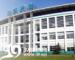• 北京双鹤药业股份有限公司
• [药企地址]北京市朝阳区望京利泽东二路1号
• [联系电话]010-64742227
• [企业简介]

双鹤研究院是北京双鹤药业股份有限公司所属的医药专业研发机构。双鹤研究院主要由双鹤药业的研发机?...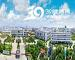• 浙江海正药业股份有限公司
• [药企地址]浙江省台州市椒江区外沙路
• [联系电话]0576-88827890,88088385,88089080,88087600
• [企业简介]

浙江海正药业股份有限公司始创于1956年，位于中国东南沿海的港口城市---浙江省台州市。目前海正已成...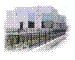• 天津力生制药股份有限公司
• [药企地址]天津市南开区黄河道 491号
• [联系电话]022-27362444
• [企业简介]

天津力生制药股份有限公司系天津市医药系统的大型企业，始建于1951年，系具有五十多年历史的天津市?...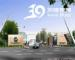• 北京紫竹药业有限公司
• [药企地址]北京朝阳区朝阳北路27号
• [联系电话]010-65483355
• [企业简介]

北京紫竹药业有限公司，是由具有三十多年历史的北京第三制药厂于2000年改制而成的一家大型综合性高?...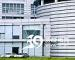• 桂林三金药业股份有限公司
• [药企地址]广西桂林市金星路一号
• [联系电话]0773-5837143 5843262 5815858 5848825
• [企业简介]

桂林三金药业股份有限公司是三金集团的核心企业，主营中成药、天然药物的研发和生产，是国内最早生...• 上海新先锋药业有限公司
• [药企地址]上海浦东张江高科技园区张江路92号
• [联系电话]021-58552452
• [企业简介]

上海医药（集团）有限公司抗生素事业部的战略定位：以抗生素为主，做精、做强、做大非专利的处方药?...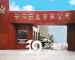• 山西云鹏制药有限公司
• [药企地址]山西省临汾市解放东路81号
• [联系电话]0357-3018036
• [企业简介]

云鹏药业有限公司是1993年由二十八集团军投资兴建军的制剂生产厂于1996年改造 成为股份制企业 ，主?...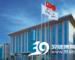• 广东华南药业有限公司
• [药企地址]广东省东莞市石龙镇西湖工业区信息产业园
• [联系电话]0769-86100999
• [企业简介]

广东华南药业集团是一家致力于人类健康产品的高新技术企业，经过近三十年的发展，已经形成为科工贸?...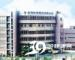• 深圳致君制药有限公司
• [药企地址]深圳市宝安区观澜高新技术产业园区
• [联系电话]0755-82433388
• [企业简介]

深圳致君制药有限公司作为国药控股旗下深圳一致药业的核心企业，其前身为深圳市制药厂，成立于1985?...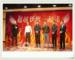• 重庆华邦制药股份有限公司
• [药企地址]重庆市渝北区人和星光大道69号
• [联系电话]023-67889222 、67886921
• [企业简介]

重庆华邦制药股份有限公司位于重庆市高新技术开发区，是一家注册资金13200万，员工近600多名，总资?...• 上海信谊药厂有限公司
• [药企地址]上海市浦东新区新金桥路 905 号
• [联系电话]021-58995818
• [企业简介]信谊是中国化学制药企业中产品最多，剂型最全的产业实体之一，集制造、销售、研发为一体的知名大型民族医药...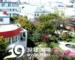• 上海中西三维药业有限公司
• [药企地址]上海市普陀区永登路50号
• [联系电话]021-62506900
• [企业简介]

上海中西三维药业有限公司由具有百年历史沉淀的上海中西药业股份有限公司和国内大型制药企业上海三?...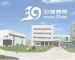• 上海禾丰制药有限公司
• [药企地址]上海市浦东金桥开发区金沪路805号
• [联系电话]021-50317200,50312222
• [企业简介]

一．企业概况

上海禾丰制药有限公司前身为上海天丰药厂，是一家具有近70年历史的优秀制...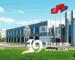• 沈阳红旗制药有限公司
• [药企地址]沈阳市浑南新区新络街6号
• [联系电话]024-23786266
• [企业简介]

始建于1964年拥有四十年企业历史的沈阳红旗制药有限公司，座落于景色秀丽的沈阳浑南新区,占地面积65...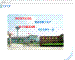• 常州制药厂有限公司
• [药企地址]常州市劳动东路518号
• [联系电话]0519-88813251
• [企业简介]

常州制药厂有限公司始建于1949年，与共和国同龄，前身为常州制药厂，2002年1月，公司完成股份制改造...

1 2 3 4 5 6 首页 上一页 下一页 末页